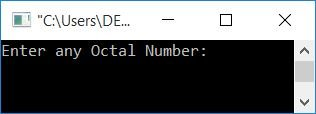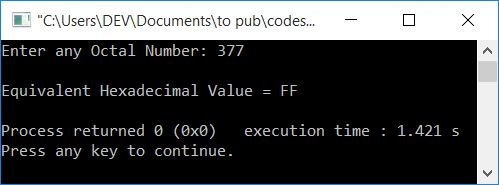# C++ Program to Convert Octal to Hexadecimal

In this article, you will learn about and get code for octal to hexadecimal conversion in C++. The program is created with and without using user-defined functions.

Before going through the program, if you are not aware of the formula or steps used for the conversion of octal to hexadecimal, you can refer to the octal to hexadecimal steps to get everything that is required during the conversion from octal to hexadecimal.

## Without Function, Octal to Hexadecimal in C++

To convert an octal number to a hexadecimal number in C++ programming, you have to ask the user to enter the octal number first. And then convert it into its equivalent hexadecimal value as shown in the program given below:

```#include<iostream>
#include<string.h>
using namespace std;
int main()
{
int octalNum, rev=0, rem, chk=0, hex=0, mul=1, i=0, k=0;
char binaryNum = "", hexnum;
cout<<"Enter any Octal Number: ";
cin>>octalNum;
while(octalNum!=0)
{
rem = octalNum%10;
if(rem>7)
{
chk++;
break;
}
rev = (rev*10) + rem;
octalNum = octalNum/10;
}
if(chk==0)
{
octalNum = rev;
while(octalNum!=0)
{
rem = octalNum%10;
switch(rem)
{
case 0: strcat(binaryNum, "000");
break;
case 1: strcat(binaryNum, "001");
break;
case 2: strcat(binaryNum, "010");
break;
case 3: strcat(binaryNum, "011");
break;
case 4: strcat(binaryNum, "100");
break;
case 5: strcat(binaryNum, "101");
break;
case 6: strcat(binaryNum, "110");
break;
case 7: strcat(binaryNum, "111");
break;
}
octalNum = octalNum/10;
}
while(binaryNum[k]!='\0')
k++;
chk=1;
k--;
while(k>=0)
{
if(binaryNum[k]=='0')
rem = 0;
else
rem = 1;
hex = hex + (rem*mul);
if(chk%4==0)
{
if(hex<10)
hexnum[i] = hex+48;
else
hexnum[i] = hex+55;
mul = 1;
hex = 0;
chk = 1;
i++;
}
else
{
mul = mul*2;
chk++;
}
k--;
}
if(chk!=1)
hexnum[i] = hex+48;
if(chk==1)
i--;
chk = 0;
for(i=i; i>=0; i--)
{
if(hexnum[i]=='0' && chk==0)
{
chk++;
continue;
}
else
cout<<hexnum[i];
}
}
else
cout<<"\nInvalid Octal Digit "<<rem;
cout<<endl;
return 0;
}```

This program was built and runs under the Code::Blocks IDE. Here is its sample run:Now input any octal number, say 377, and press the ENTER key to see its equivalent hexadecimal value as shown in the output given below:This program converts octal to binary and then binary to hexadecimal.

You can also approach the second way, which is octal to decimal and then decimal to hexadecimal. Here, the first way is used because the second way is a little easier to create and understand. So you can follow both the article and create it yourself.

## Octal to Hexadecimal using a Function in C++

This program does the same job as the previous program. The only difference is that it is created using the user-defined function octalToHexa().

```#include<iostream>
#include<string.h>
int reverseNum(int);
void octalToHexa(int);
static int chk, i;
char hexnum;
using namespace std;
int main()
{
int octalNum;
cout<<"Enter any Octal Number: ";
cin>>octalNum;
octalToHexa(octalNum);
if(chk!=0)
{
chk = 0;
for(i=i; i>=0; i--)
{
if(hexnum[i]=='0' && chk==0)
{
chk++;
continue;
}
else
cout<<hexnum[i];
}
}
else
cout<<"\nInvalid Octal Digit!";
cout<<endl;
return 0;
}
void octalToHexa(int octalNum)
{
int rev=0, rem, hex=0, mul=1, k=0;
char binnum = "";
rev = reverseNum(octalNum);
if(rev!=0)
{
octalNum = rev;
while(octalNum!=0)
{
rem = octalNum%10;
switch(rem)
{
case 0: strcat(binnum, "000");
break;
case 1: strcat(binnum, "001");
break;
case 2: strcat(binnum, "010");
break;
case 3: strcat(binnum, "011");
break;
case 4: strcat(binnum, "100");
break;
case 5: strcat(binnum, "101");
break;
case 6: strcat(binnum, "110");
break;
case 7: strcat(binnum, "111");
break;
}
octalNum = octalNum/10;
}
while(binnum[k]!='\0')
k++;
chk=1;
k--;
while(k>=0)
{
if(binnum[k]=='0')
rem = 0;
else
rem = 1;
hex = hex + (rem*mul);
if(chk%4==0)
{
if(hex<10)
hexnum[i] = hex+48;
else
hexnum[i] = hex+55;
mul = 1;
hex = 0;
chk = 1;
i++;
}
else
{
mul = mul*2;
chk++;
}
k--;
}
if(chk!=1)
hexnum[i] = hex+48;
if(chk==1)
i--;
}
else
chk=0;
}
int reverseNum(int octalNum)
{
int rem, rev=0;
while(octalNum!=0)
{
rem = octalNum%10;
if(rem>7)
return 0;
rev = (rev*10) + rem;
octalNum = octalNum/10;
}
return rev;
}```

It produces the same output as the previous program.

#### The same program in different languages

C++ Quiz

« Previous Program Next Program »